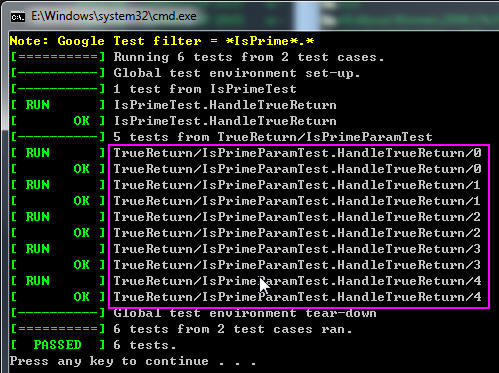# 二、旧的方案

// Returns true iff n is a prime number.
bool IsPrime(int n)
{
// Trivial case 1: small numbers
if (n <= 1) return false;

// Trivial case 2: even numbers
if (n % 2 == 0) return n == 2;

// Now, we have that n is odd and n >= 3.

// Try to divide n by every odd number i, starting from 3
for (int i = 3; ; i += 2) {
// We only have to try i up to the squre root of n
if (i > n/i) break;

// Now, we have i <= n/i < n.
// If n is divisible by i, n is not prime.
if (n % i == 0) return false;
}
// n has no integer factor in the range (1, n), and thus is prime.
return true;
}

TEST(IsPrimeTest, HandleTrueReturn)
{
EXPECT_TRUE(IsPrime(3));
EXPECT_TRUE(IsPrime(5));
EXPECT_TRUE(IsPrime(11));
EXPECT_TRUE(IsPrime(23));
EXPECT_TRUE(IsPrime(17));
}

# 三、使用参数化后的方案

1. 告诉gtest你的参数类型是什么

class IsPrimeParamTest : public::testing::TestWithParam<int>
{

};

2. 告诉gtest你拿到参数的值后，具体做些什么样的测试

TEST_P(IsPrimeParamTest, HandleTrueReturn)
{
int n =  GetParam();
EXPECT_TRUE(IsPrime(n));
}

3. 告诉gtest你想要测试的参数范围是什么

使用INSTANTIATE_TEST_CASE_P这宏来告诉gtest你要测试的参数范围：

INSTANTIATE_TEST_CASE_P(TrueReturn, IsPrimeParamTest, testing::Values(3, 5, 11, 23, 17));

 Range(begin, end[, step]) 范围在begin~end之间，步长为step，不包括end Values(v1, v2, ..., vN) v1,v2到vN的值 ValuesIn(container) and ValuesIn(begin, end) 从一个C类型的数组或是STL容器，或是迭代器中取值 Bool() 取false 和 true 两个值 Combine(g1, g2, ..., gN) 这个比较强悍，它将g1,g2,...gN进行排列组合，g1,g2,...gN本身是一个参数生成器，每次分别从g1,g2,..gN中各取出一个值，组合成一个元组(Tuple)作为一个参数。 说明：这个功能只在提供了头的系统中有效。gtest会自动去判断是否支持tr/tuple，如果你的系统确实支持，而gtest判断错误的话，你可以重新定义宏GTEST_HAS_TR1_TUPLE=1。

# 四、参数化后的测试案例名prefix/test_case_name.test.name/index

# 五、类型参数化

gtest还提供了应付各种不同类型的数据时的方案，以及参数化类型的方案。我个人感觉这个方案有些复杂。首先要了解一下类型化测试，就用gtest里的例子了。

template <typename T>
class FooTest : public testing::Test {
public:typedef std::list<T> List;
static T shared_;
T value_;
};

typedef testing::Types<char, int, unsigned int> MyTypes;
TYPED_TEST_CASE(FooTest, MyTypes);

TYPED_TEST(FooTest, DoesBlah) {
// Inside a test, refer to the special name TypeParam to get the type
// parameter.  Since we are inside a derived class template, C++ requires
// us to visit the members of FooTest via 'this'.
TypeParam n = this->value_;

// To visit static members of the fixture, add the 'TestFixture::'
// prefix.
n += TestFixture::shared_;

// To refer to typedefs in the fixture, add the 'typename TestFixture::'
// prefix.  The 'typename' is required to satisfy the compiler.
typename TestFixture::List values;
values.push_back(n);}

template <typename T>
class FooTest : public testing::Test {};

TYPED_TEST_CASE_P(FooTest);

TYPED_TEST_P(FooTest, DoesBlah) {
// Inside a test, refer to TypeParam to get the type parameter.
TypeParam n = 0;}

TYPED_TEST_P(FooTest, HasPropertyA) {}

REGISTER_TYPED_TEST_CASE_P(FooTest, DoesBlah, HasPropertyA);

typedef testing::Types<char, int, unsigned int> MyTypes;
INSTANTIATE_TYPED_TEST_CASE_P(My, FooTest, MyTypes);

# 六、总结

gtest为我们提供的参数化测试的功能给我们的测试带来了极大的方便，使得我们可以写更少更优美的代码，完成多种参数类型的测试案例。

posted @ 2019-01-06 19:17  克拉默与矩阵  阅读(...)  评论(...编辑  收藏